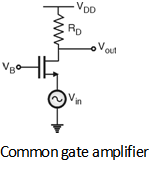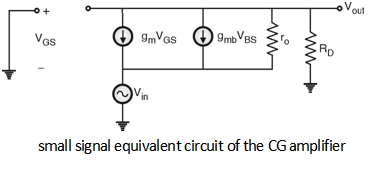Home > Analog CMOS Design > MOSFET Amplifiers > Common Gate Amplifier

Common Gate Amplifier :

In common source amplifier and source follower circuits, the input signal is applied to the gate of a MOSFET. It is also possible to apply the input signal to the source terminal by keeping common gate terminal. This type of amplifier is called as common gate amplifier.

Figure below shows the CG amplifier in which the input signal is sensed at the source terminal and the output is produced at the drain terminal. The gate terminal is connected to VB i.e. dc potential which will maintain the proper operating conditions.Figure below shows the small signal equivalent circuit of the CG amplifier.By analizing the small signal equivalent circuit, the voltage gain of CG amplifier is given by,

Av = = gm RD

The important point is the gain is positive, further the input impedance is given by which shows that the input impedance of common gate amplifier is relatively low. Furthermore, the input impedance of of common gate stage is relatively low only if the load resistance connected to the drain is small.